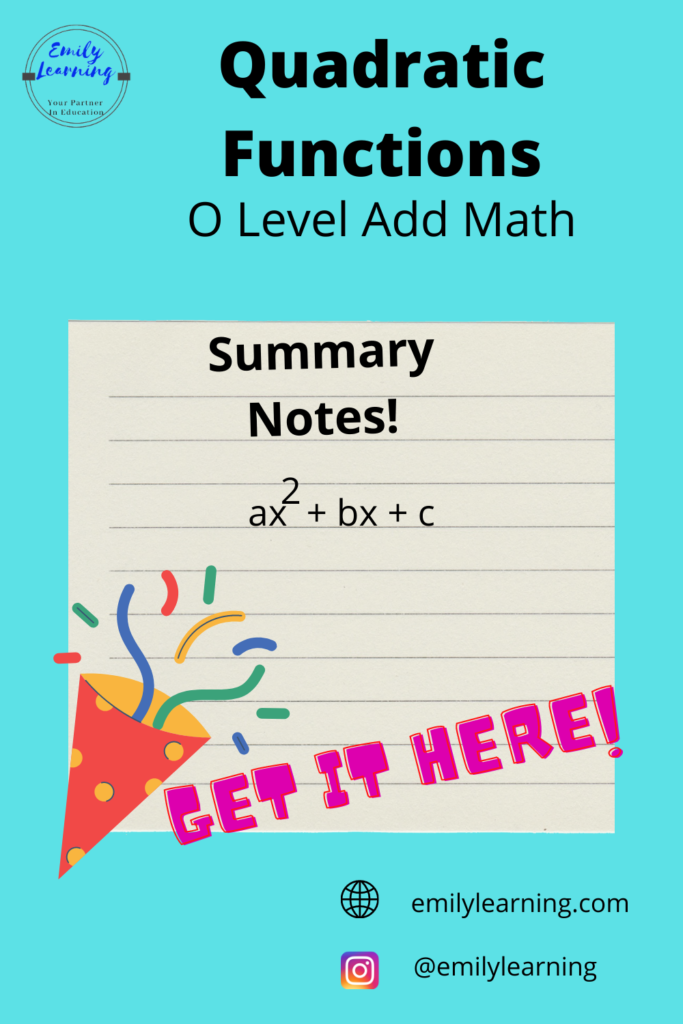Here, you’ll find a summary of what’s tested in the Quadratic function for O Level Additional Mathematics. If you are interested to know what’s in the syllabus for quadratic functions, I gave details in this post here.

Quadratic function is one of the first few topics students learn in O Level Additional Mathematics. This topic revolves around the quadratic expression ax2 + bx +c, its graph, finding the number of solutions (when it’s an equation), and solving it as an inequality. Solving quadratic equations is something that you learned in elementary Mathematics, and is assumed knowledge for this chapter.

So now, let’s summarize the concepts for quadratic functions:

•  Completing the square:
• this involves manipulating the equation from ax2+bx+c into a(x+h)2 + g
• if a >0, the curve has minimum point with coordinates (-h, g)
• if a<0, the curve has a maximum point with coordinates (-h, g)
• Discriminant of quadratic functions: b2 – 4ac
• value of discriminant determines the number of real distinct roots of a quadratic equation
• if  b2 – 4ac > 0, there are 2 distinct real roots
• if  b2 – 4ac = 0, there are equal real roots
• if  b2 – 4ac < 0, there are no real roots
• If you have a curve of the equation, y = f(x), and a line of equation y = g(x), you’ll first need to equate f(x) to g(x) and manipulate it until it becomes the form ax2+bx+c (if you can’t make it is not a quadratic form, then it’s not a discriminant question)
• if  b2 – 4ac > 0, line cuts curve at 2 distinct point
• if  b2 – 4ac = 0, line is a tangent to the curve
• if  b2 – 4ac < 0, line and curve do not intersect